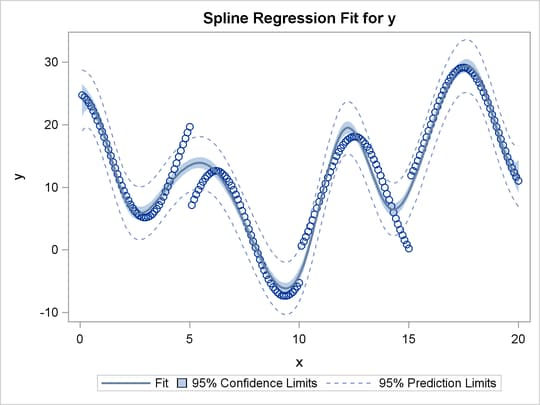Using Splines and Knots

This section illustrates some properties of splines. Splines are curves, and they are usually required to be continuous and smooth. Splines are usually defined as piecewise polynomials of degree n with function values and first n – 1 derivatives that agree at the points where they join. The abscissa or X-axis values of the join points are called knots. The term spline is also used for polynomials (splines with no knots) and piecewise polynomials with more than one discontinuous derivative. Splines with no knots are generally smoother than splines with knots, which are generally smoother than splines with multiple discontinuous derivatives. Splines with few knots are generally smoother than splines with many knots; however, increasing the number of knots usually increases the fit of the spline function to the data. Knots give the curve freedom to bend to more closely follow the data. See Smith (1979) for an excellent introduction to splines.

In this section, an artificial data set is created with a variable y that is a discontinuous function of x. (See Figure 97.20.) Notice that the function has four unconnected parts, each of which is a curve. Notice too that there is an overall quadratic trend—that is, ignoring the shapes of the individual curves, at first the y values tend to decrease as x increases, then y values tend to increase. While these artificial data are clearly not realistic, their distinct pattern helps illustrate how splines work. The following statements create the data set, fit a simple linear regression model, and produce Figure 97.19 through Figure 97.20:

title 'An Illustration of Splines and Knots';

* Create in y a discontinuous function of x.;

data a;
x = -0.000001;
do i = 0 to 199;
if mod(i, 50) = 0 then do;
c = ((x / 2) - 5)**2;
if i = 150 then c = c + 5;
y = c;
end;
x = x + 0.1;
y = y - sin(x - c);
output;
end;
run;

ods graphics on;

title2 'A Linear Regression Fit';
proc transreg data=a plots=scatter rsquare;
model identity(y) = identity(x);
run;

The R square for the linear regression is 0.1006. The linear fit results in Figure 97.19 show the predicted values of y given x. It can clearly be seen in Figure 97.19 that the linear regression model is not appropriate for these data.

Figure 97.19: A Linear Regression Fit

 An Illustration of Splines and Knots A Linear Regression Fit

The TRANSREG Procedure

The TRANSREG Procedure Hypothesis Tests for Identity(y)

 R-Square 0.1006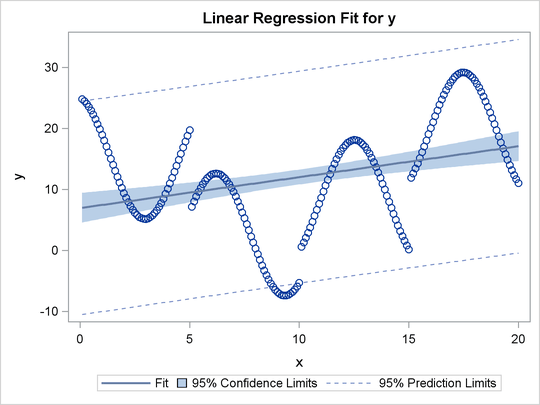Figure 97.20: The Original Scatter Plot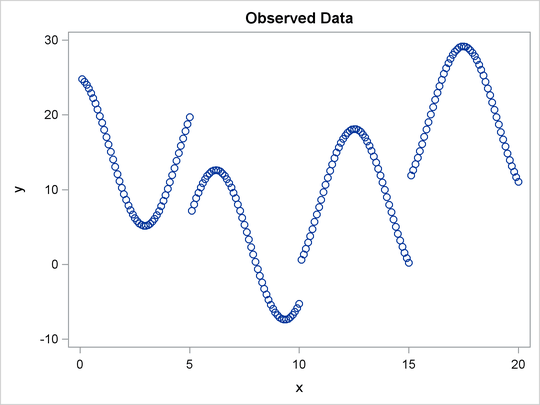The next PROC TRANSREG step finds a degree-two spline transformation with no knots, which is a quadratic polynomial. The spline is a weighted sum of a single constant, a single straight line, and a single quadratic curve. The following statements perform the quadratic analysis and produce Figure 97.21:

proc transreg data=A;
model identity(y)=spline(x / degree=2);
run;

The R square in Figure 97.21 increases from 0.10061, which is the linear fit value from before, to 0.40720. The plot shows that the quadratic regression function does not fit any of the individual curves well, but it does follow the overall trend in the data. Since the overall trend is quadratic, if you were to fit a degree-three spline with no knots (not shown) would increase R square by only a small amount.

Figure 97.21: A Quadratic Polynomial Fit

 An Illustration of Splines and Knots A Quadratic Polynomial Fit

The TRANSREG Procedure

TRANSREG MORALS Algorithm Iteration History for Identity(y)
Iteration
Number
Average
Change
Maximum
Change
R-Square Criterion
Change
Note
1 0.82127 2.77121 0.10061
2 0.00000 0.00000 0.40720 0.30659 Converged

 Algorithm converged.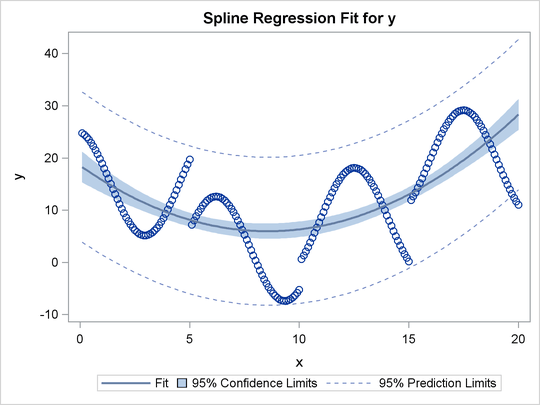The next step uses the default degree of three, for a piecewise cubic polynomial, and requests knots at the known break points, x=5, 10, and 15. This requests a spline that is continuous, has continuous first and second derivatives, and has a third derivative that is discontinuous at 5, 10, and 15. The spline is a weighted sum of a single constant, a single straight line, a single quadratic curve, a cubic curve for the portion of x less than 5, a different cubic curve for the portion of x between 5 and 10, a different cubic curve for the portion of x between 10 and 15, and another cubic curve for the portion of x greater than 15. The following statements fit the spline model and produce Figure 97.22:

title2 'A Cubic Spline Fit with Knots at X=5, 10, 15';

proc transreg data=a;
model identity(y) = spline(x / knots=5 10 15);
run;

The new R square in Figure 97.22 is 0.61730. The plot shows that the spline is less smooth than the quadratic polynomial and follows the data more closely than the quadratic polynomial.

Figure 97.22: A Cubic Spline Fit

 An Illustration of Splines and Knots A Cubic Spline Fit with Knots at X=5, 10, 15

The TRANSREG Procedure

TRANSREG MORALS Algorithm Iteration History for Identity(y)
Iteration
Number
Average
Change
Maximum
Change
R-Square Criterion
Change
Note
1 0.85367 3.88449 0.10061
2 0.00000 0.00000 0.61730 0.51670 Converged

 Algorithm converged.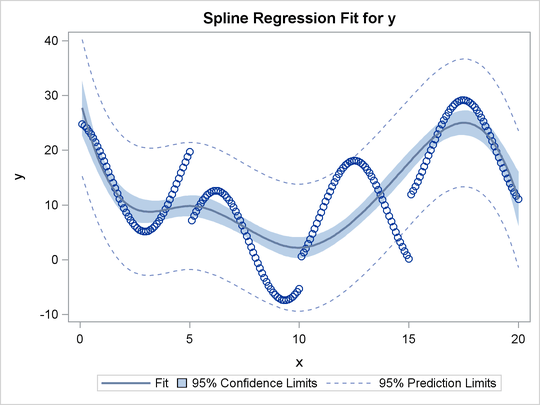The same model could be fit with a DATA step and PROC REG, as follows:

data b;             /* A is the data set used by transreg */
set a(keep=x y);
x1=x;                       /* x                       */
x2=x**2;                    /* x squared               */
x3=x**3;                    /* x cubed                 */
x4=(x> 5)*((x-5)**3);       /* change in x**3 after  5 */
x5=(x>10)*((x-10)**3);      /* change in x**3 after 10 */
x6=(x>15)*((x-15)**3);      /* change in x**3 after 15 */
run;

proc reg;
model y=x1-x6;
run; quit;

The output from these previous statements is not displayed. The assignment statements and comments show how you can construct terms that can be used to fit the same model.

In the next step, each knot is repeated three times, so the first, second, and third derivatives are discontinuous at x=5, 10, and 15, but the spline is continuous at the knots. The spline is a weighted sum of the following:

• a single constant

• a line for the portion of x less than 5

• a quadratic curve for the portion of x less than 5

• a cubic curve for the portion of x less than 5

• a different line for the portion of x between 5 and 10

• a different quadratic curve for the portion of x between 5 and 10

• a different cubic curve for the portion of x between 5 and 10

• a different line for the portion of x between 10 and 15

• a different quadratic curve for the portion of x between 10 and 15

• a different cubic curve for the portion of x between 10 and 15

• another line for the portion of x greater than 15

• another quadratic curve for the portion of x greater than 15

• another cubic curve for the portion of x greater than 15

The spline is continuous since there is not a separate constant or separate intercept in the formula for the spline for each knot. The following statements perform this analysis and produce Figure 97.23:

title3 'First - Third Derivatives Discontinuous at X=5, 10, 15';

proc transreg data=a;
model identity(y) = spline(x / knots=5 5 5 10 10 10 15 15 15);
run;

Now the R square in Figure 97.23 is 0.95542, and the spline closely follows the data, except at the knots.

Figure 97.23: Spline with Discontinuous Derivatives

 An Illustration of Splines and Knots A Cubic Spline Fit with Knots at X=5, 10, 15 First - Third Derivatives Discontinuous at X=5, 10, 15

The TRANSREG Procedure

TRANSREG MORALS Algorithm Iteration History for Identity(y)
Iteration
Number
Average
Change
Maximum
Change
R-Square Criterion
Change
Note
1 0.92492 3.50038 0.10061
2 0.00000 0.00000 0.95542 0.85481 Converged

 Algorithm converged.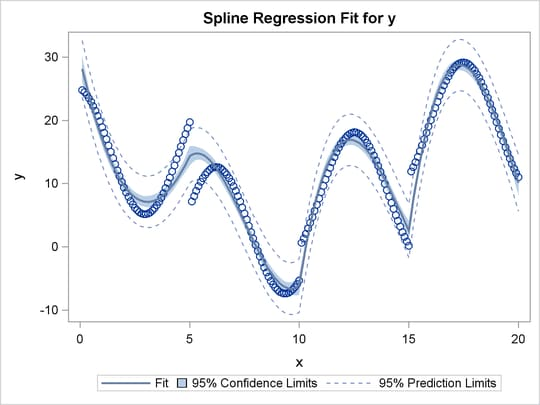The same model could be fit with a DATA step and PROC REG, as follows:

data b;
set a(keep=x y);
x1=x;                        /* x                       */
x2=x**2;                     /* x squared               */
x3=x**3;                     /* x cubed                 */
x4=(x>5)   * (x- 5);         /* change in x    after  5 */
x5=(x>10)  * (x-10);         /* change in x    after 10 */
x6=(x>15)  * (x-15);         /* change in x    after 15 */
x7=(x>5)   * ((x-5)**2);     /* change in x**2 after  5 */
x8=(x>10)  * ((x-10)**2);    /* change in x**2 after 10 */
x9=(x>15)  * ((x-15)**2);    /* change in x**2 after 15 */
x10=(x>5)  * ((x-5)**3);     /* change in x**3 after  5 */
x11=(x>10) * ((x-10)**3);    /* change in x**3 after 10 */
x12=(x>15) * ((x-15)**3);    /* change in x**3 after 15 */
run;

proc reg;
model y=x1-x12;
run; quit;

The output from these previous statements is not displayed. The assignment statements and comments show how you can construct terms that can be used to fit the same model.

Each knot is repeated four times in the next step. Now the spline function is discontinuous at the knots, and it can follow the data more closely. The following statements perform this analysis and produce Figure 97.24:

title3 'Discontinuous Function and Derivatives';

proc transreg data=a;
model identity(y) = spline(x / knots=5 5 5 5 10 10 10 10
15 15 15 15);
run;

Now the R square in Figure 97.24 is 0.99254. In this step, each separate curve is approximated by a cubic polynomial (with no knots within the separate polynomials). (Note, however, that the separate functions are connected in the plot, because PROC TRANSREG cannot currently produce separate functions for a model like this. Usually, you would use a CLASS variable to get separate functions.)

Figure 97.24: Discontinuous Spline Fit

 An Illustration of Splines and Knots A Cubic Spline Fit with Knots at X=5, 10, 15 Discontinuous Function and Derivatives

The TRANSREG Procedure

TRANSREG MORALS Algorithm Iteration History for Identity(y)
Iteration
Number
Average
Change
Maximum
Change
R-Square Criterion
Change
Note
1 0.90271 3.29184 0.10061
2 0.00000 0.00000 0.99254 0.89193 Converged

 Algorithm converged.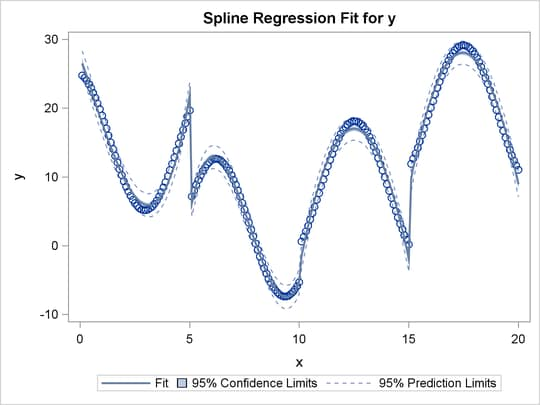To solve this problem with a DATA step and PROC REG, you would need to create all of the variables in the preceding DATA step (the B data set for the piecewise polynomial with discontinuous third derivatives), plus the following three variables:

x13=(x >  5);   /* intercept change after  5 */
x14=(x > 10);   /* intercept change after 10 */
x15=(x > 15);   /* intercept change after 15 */

The next two examples use the NKNOTS= t-option to specify the number of knots but not their location. NKNOTS=4 places knots at the quintiles, whereas NKNOTS=9 places knots at the deciles. The spline and its first two derivatives are continuous. The following statements produce Figure 97.25 and Figure 97.26:

title3 'Four Knots';

proc transreg data=a;
model identity(y) = spline(x / nknots=4);
run;

title3 'Nine Knots';

proc transreg data=a;
model identity(y) = spline(x / nknots=9);
run;

The R-square values displayed in Figure 97.25 and Figure 97.26 are 0.74450 and 0.95256, respectively. Even though the knots are not optimally placed, the spline can closely follow the data with NKNOTS=9.

Figure 97.25: Spline Fit with Knots at the Quintiles

 An Illustration of Splines and Knots A Cubic Spline Fit with Knots at X=5, 10, 15 Four Knots

The TRANSREG Procedure

TRANSREG MORALS Algorithm Iteration History for Identity(y)
Iteration
Number
Average
Change
Maximum
Change
R-Square Criterion
Change
Note
1 0.90305 4.46027 0.10061
2 0.00000 0.00000 0.74450 0.64389 Converged

 Algorithm converged.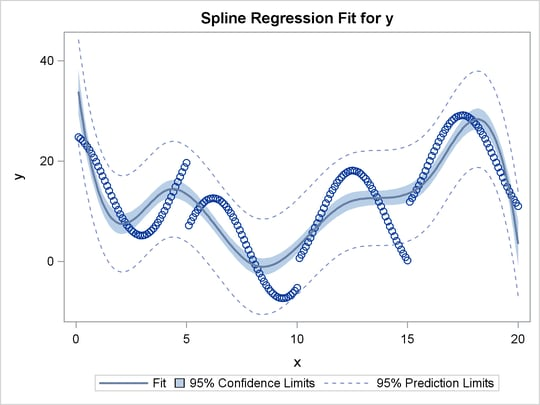Figure 97.26: Spline Fit with Knots at the Deciles

 An Illustration of Splines and Knots A Cubic Spline Fit with Knots at X=5, 10, 15 Nine Knots

The TRANSREG Procedure

TRANSREG MORALS Algorithm Iteration History for Identity(y)
Iteration
Number
Average
Change
Maximum
Change
R-Square Criterion
Change
Note
1 0.94832 3.03488 0.10061
2 0.00000 0.00000 0.95256 0.85196 Converged

 Algorithm converged.